The `scatterD3` package provides an HTML widget based on the `htmlwidgets` package and allows to produce interactive scatterplots by using the `d3.js` javascript visualization library.

## Basic scatterplot

Starting with the sample `mtcars` dataset, we can produce a basic scatterplot with the following command :

``````library(scatterD3)
scatterD3(x = mtcars\$wt, y = mtcars\$mpg)
``````

You can pass data arguments as vectors, like above, but you can also give a data frame as `data` argument and then provide variable names which will be evaluated inside this data frame :

``````scatterD3(data = mtcars , x = wt, y = mpg)
``````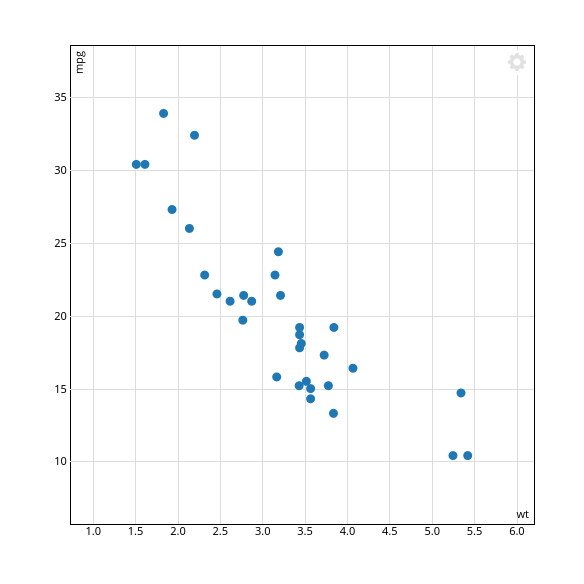This will display a simple visualization with the given variables as `x` and `y` axis. There are several interactive features directly available :

• you can zoom in and out with the mouse wheel while the mouse cursor is on the plot
• you can pan the plot by dragging with your mouse
• by hovering over a point, you can display a small tooltip window giving the `x` and `y` values

You can customize the points size with the `point_size` parameter, their global opacity with `point_opacity`, and you can force the plot to have a 1:1 fixed aspect ratio with `fixed = TRUE`. You can also manually specify the points color with the `colors` argument

``````scatterD3(data = mtcars, x = wt, y = mpg,
point_size = 35, point_opacity = 0.5, fixed = TRUE,
colors = "#A94175")
``````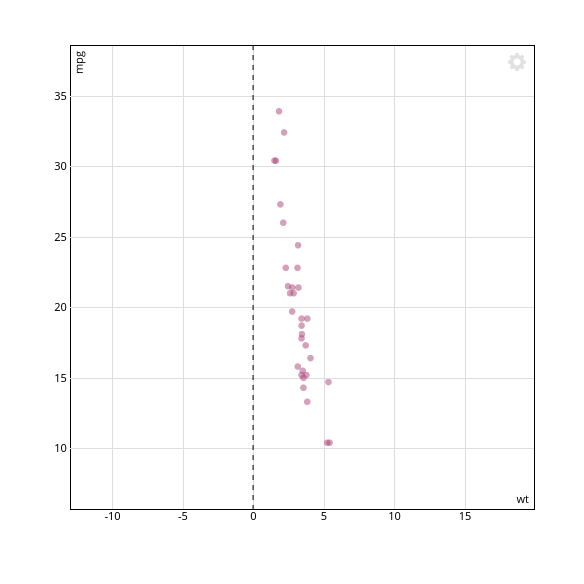You can change size and opacity of points when hovering with the `hover_size` and `hover_opacity` settings :

``````scatterD3(data = mtcars, x = wt, y = mpg,
point_size = 100, point_opacity = 0.5,
hover_size = 4, hover_opacity = 1)
``````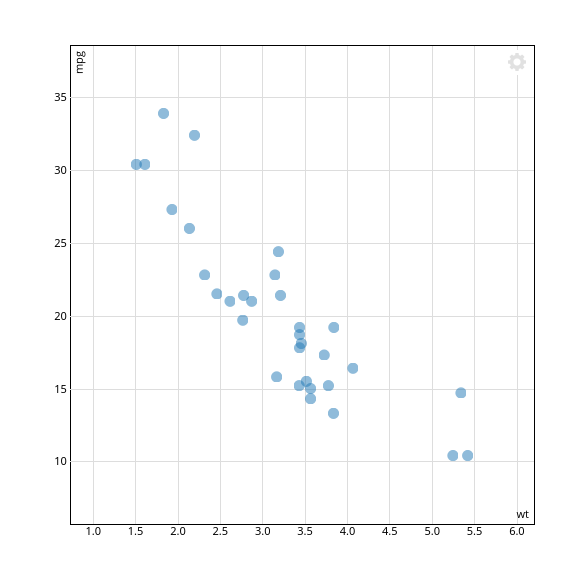## Categorical `x` and `y`

If the `x` or `y` variable is not numeric or is a factor, then an ordinal scale is used for the corresponding axis. Note that zooming is then not possible along this axis.

``````mtcars\$cyl_fac <- paste(mtcars\$cyl, "cylinders")
scatterD3(data = mtcars, x = cyl_fac, y = mpg)
``````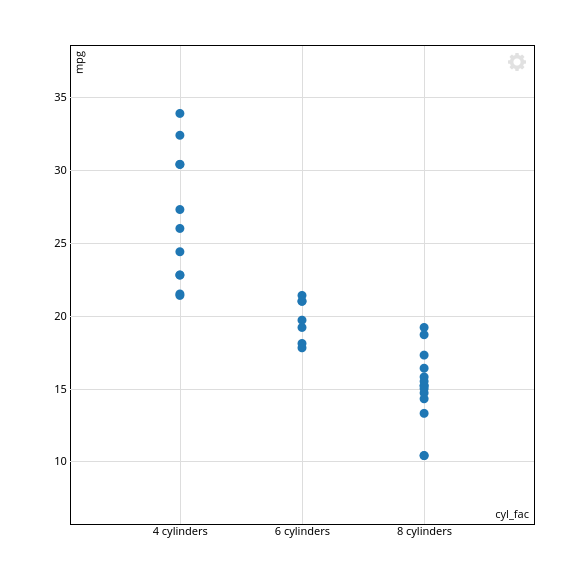You can use the `left_margin` argument when using a categorical `y` variable if the axis labels are not entirely visible :

``````scatterD3(data = mtcars, x = wt, y = cyl_fac, left_margin = 80)
``````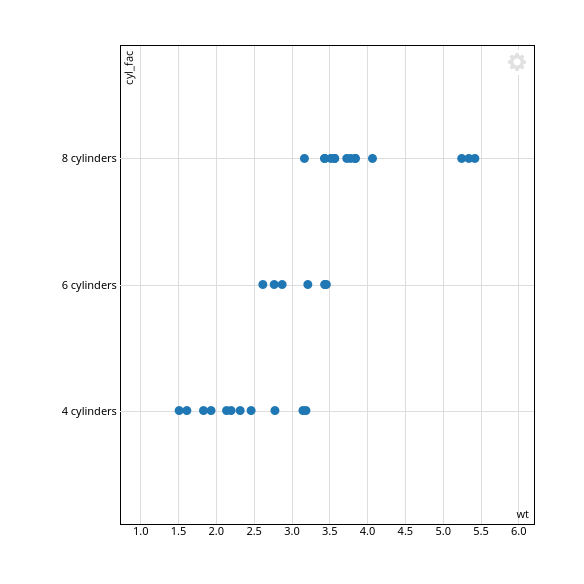## Point labels

You can add text labels to the points by passing a character vector to the `lab` parameter. Labels size are controlled by the `labels_size` parameter.

``````mtcars\$names <- rownames(mtcars)
scatterD3(data = mtcars, x = wt, y = mpg, lab = names, labels_size = 9)
``````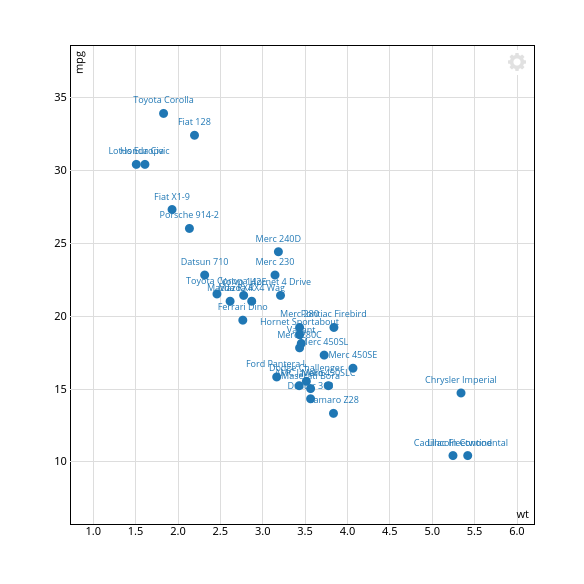Note that text labels are fully movable : click and drag a label with your mouse to place it where you want. Custom positions are preserved while zooming/panning.

## Mapping colors, symbols, size and opacity to variables

By passing vectors to the `col_var` and/or `symbol_var` arguments, you can map points colors and symbols to other variables.

``````scatterD3(data = mtcars, x = wt, y = mpg, col_var = cyl, symbol_var = gear)
``````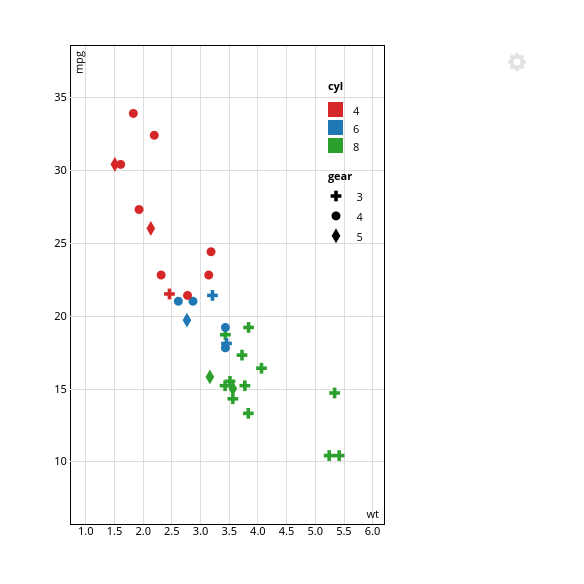A legend is then automatically added. You can manually specify its width with the `legend_width` argument. Use `legend_width = 0` to disable it entirely.

Note that when hovering over a legend item with your mouse, the corresponding points are highlighted. Also note that the mapped variables values are automatically added to the default tooltips.

You can also map symbol sizes with a variable with the `size_var` argument. `size_range` allows to customize the sizes range :

``````scatterD3(data = mtcars, x = wt, y = mpg, col_var = cyl, size_var = hp,
size_range = c(10,1000), point_opacity = 0.7)
``````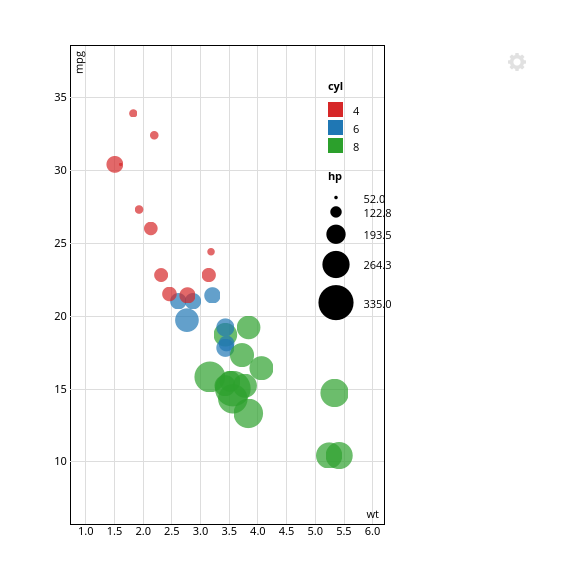You can specify custom colors by passing a vector of hexadecimal strings to the `colors` argument. If the vector is named, then the colors will be associated with their names within `col_var`.

``````scatterD3(data = mtcars, x = wt, y = mpg, col_var = cyl,
colors = c("4" = "#ECD078", "8" = "#C02942", "6" = "#53777A"))
``````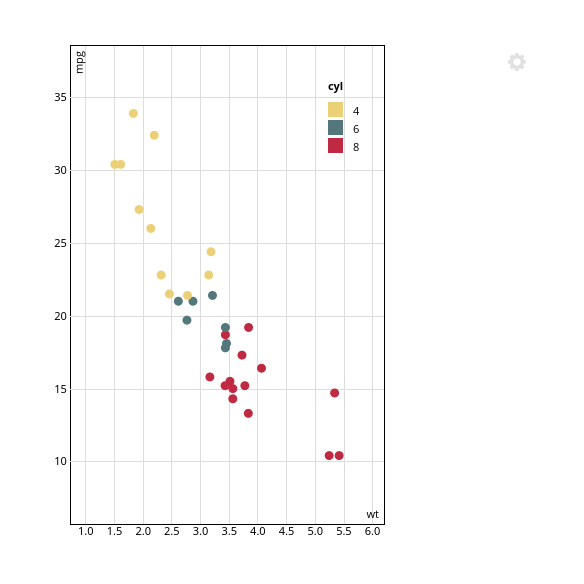If `col_var` is numeric, not a factor, and has more than 6 unique values, it is considered as continuous, and drawn accordingly using the Veridis d3 interpolator.

``````scatterD3(data = mtcars, x = wt, y = mpg, col_var = disp)
``````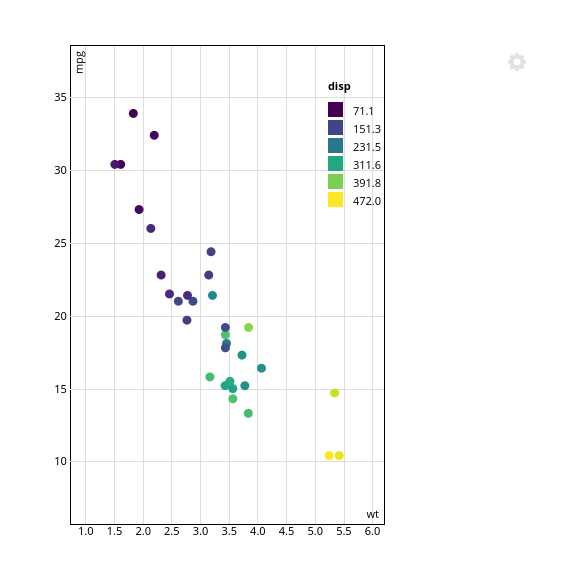In this case, any `colors` argument is ignored. You can force `col_var` to be considered as continuous with `col_continuous = TRUE`.

You can also use the `opacity_var` argument to map point opacity to a variable. Note that for now no legend for opacity is added, though.

``````scatterD3(data=mtcars, x=mpg, y=wt, opacity_var = drat)
``````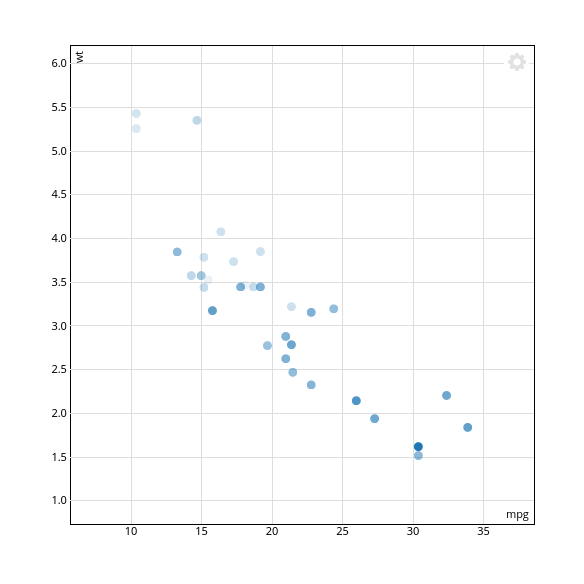In addition to your data points, you can add to your scatterplot. This is done vy passing a data frame to the `lines` argument. This data frame must have at least two columns called `slope` and `intercept`, and as many rows as lines you want to draw.

For example, if you want to add a 1:1 line :

``````scatterD3(data = mtcars, x = wt, y = mpg,
lines = data.frame(slope = -5.344, intercept = 37.285))
``````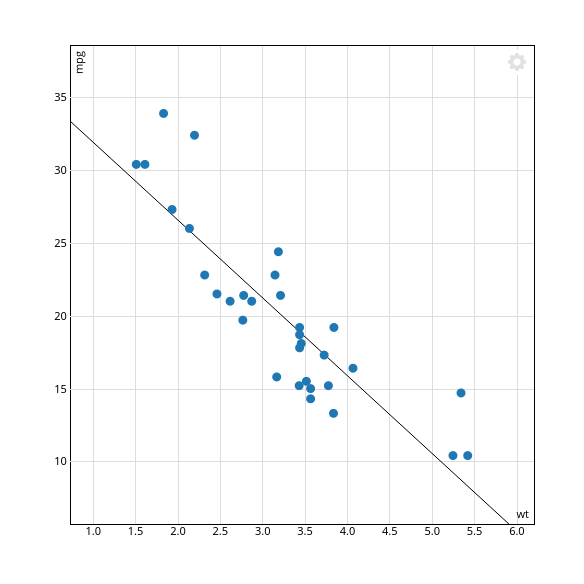You can style your lines by adding `stroke`, `stroke_width` and `stroke_dasharray` columns. These columns values will be added as corresponding styles to the generated SVG line. So if you want a wide dashed red horizontal line :

``````scatterD3(data = mtcars, x = wt, y = mpg,
lines = data.frame(slope = 0,
intercept = 30,
stroke = "red",
stroke_width = 5,
stroke_dasharray = "10,5"))
``````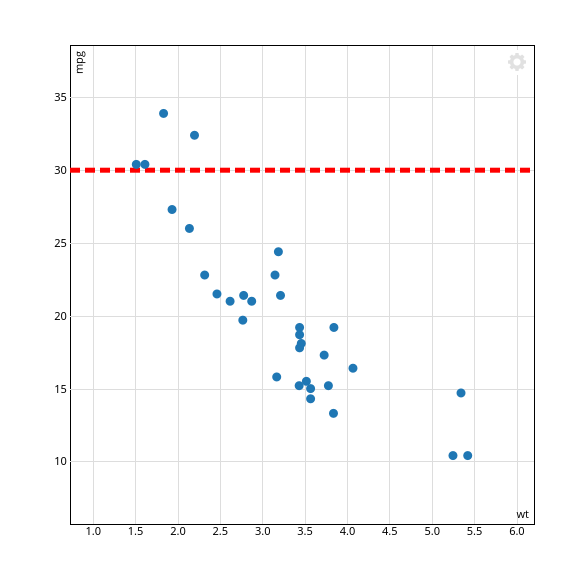If you want to draw a vertical line, pass the `Inf` value to `slope`. The value of `intercept` is then interpreted as the intercept along the x axis.

By default, if no `lines` argument is provided two dashed horizontal and vertical lines are drawn through the origin, which is equivalent to :

``````scatterD3(data = mtcars, x = wt, y = mpg, fixed = TRUE,
lines = data.frame(slope = c(0, Inf),
intercept = c(0, 0),
stroke = "#000",
stroke_width = 1,
stroke_dasharray = 5))
``````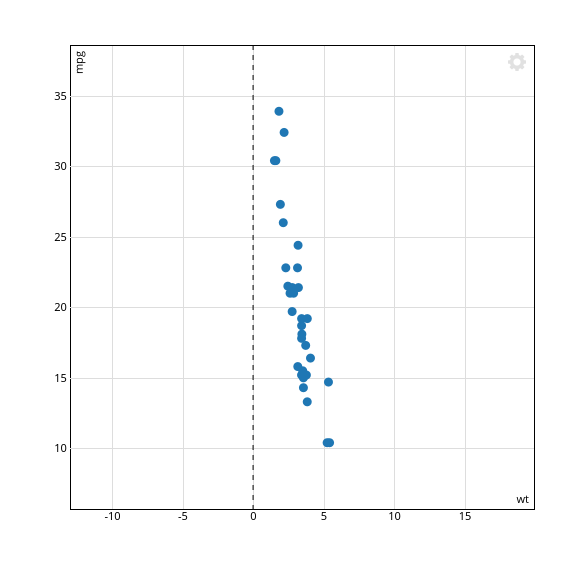## Scales, axes and legend

The `x_log` and `y_log` arguments allow to use logarithmic scales on the `x` and `y` values. Note that there must not be any value inferior or equal to zero in this case :

``````scatterD3(data = mtcars, x = wt, y = mpg, col_var = cyl,
x_log = TRUE, y_log = TRUE)
``````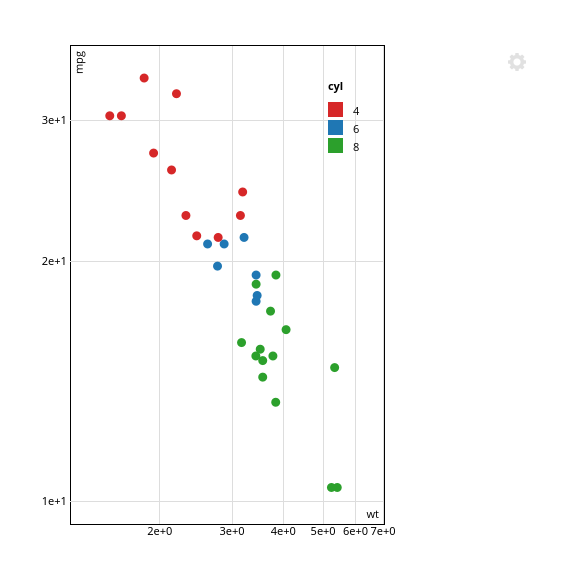You can manually specify the `x` or `y` axis limits with the `xlim` and `ylim` arguments :

``````scatterD3(data = mtcars, x = wt, y = mpg, xlim=c(0,10), ylim=c(10,35))
``````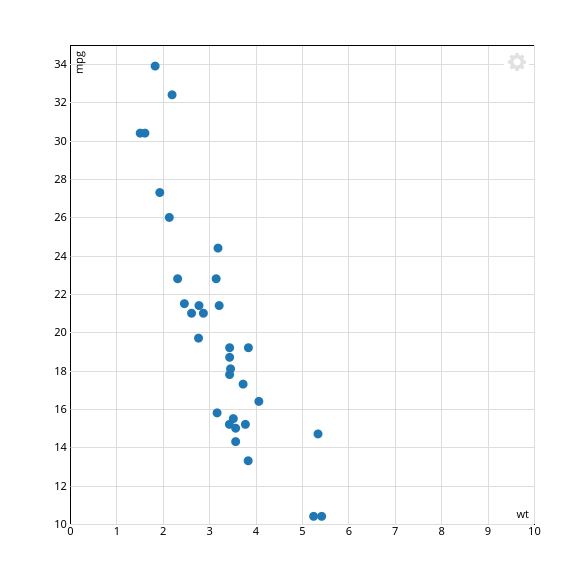You can customize the value of the axes and legend labels with `xlab`, `ylab`, `col_lab`, `symbol_lab` and `size_lab` :

``````scatterD3(data = mtcars, x = wt, y = mpg, col_var = cyl, symbol_var = gear,
xlab = "Weight", ylab = "Mpg", col_lab = "Cylinders", symbol_lab = "Gears")
``````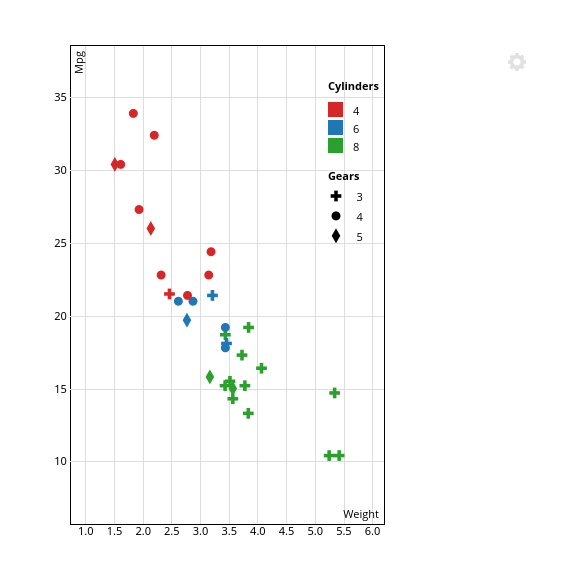Note that default tooltips are updated accordingly.

You can also change the font size of axes and legend text with `axes_font_size` and `legend_font_size` :

``````scatterD3(data = mtcars, x = wt, y = mpg, col_var = cyl,
xlab = "Weight", ylab = "Mpg",
axes_font_size = "120%",
legend_font_size = "14px")
``````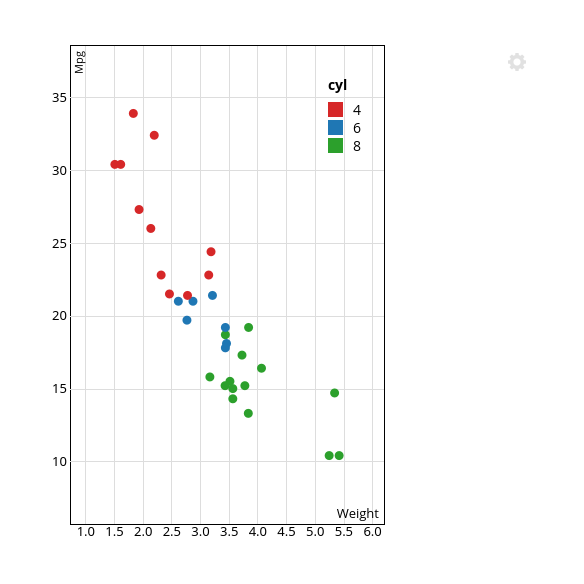You can provide any CSS compatible value, wether a fixed size such as `2em` or a relative one like `95%`.

If the left plot margin is not big enough and your y axis labels are truncated, you can adjust it with the `left_margin` argument :

``````scatterD3(data = mtcars, x = wt, y = mpg, col_var = cyl,
left_margin = 80)
``````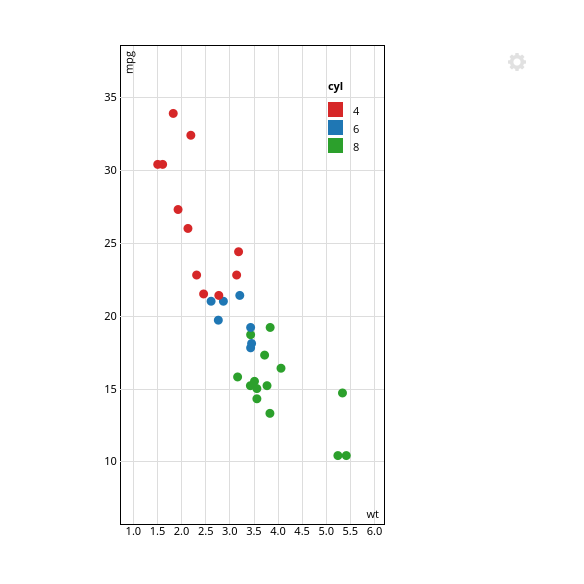## Caption

You can add an optional caption to your plot, which will be shown when clicking on a “info sign” icon in the top right of your plot.

To do so, use the `caption` argument with either a single character string :

``````scatterD3(data = mtcars, x = wt, y = mpg, col_var = cyl,
caption = "Lorem ipsum dolor sit amet, consectetur adipiscing elit. Nullam aliquam egestas pretium. Donec auctor semper vestibulum. Phasellus in tempor lacus. Maecenas vehicula, ipsum id malesuada placerat, diam lorem aliquet lectus, non lacinia quam leo quis eros.")
``````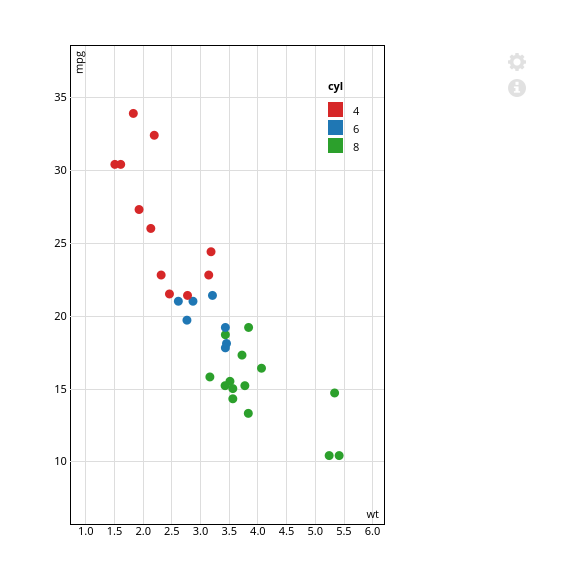Or a list with the `title`, `subtitle` and `text` elements :

``````scatterD3(data = mtcars, x = wt, y = mpg, col_var = cyl,
caption = list(title = "Caption title",
subtitle = "Caption subtitle",
text = "Lorem ipsum dolor sit amet, consectetur adipiscing elit. Nullam aliquam egestas pretium. Donec auctor semper vestibulum. Phasellus in tempor lacus. Maecenas vehicula, ipsum id malesuada placerat, diam lorem aliquet lectus, non lacinia quam leo quis eros."))
``````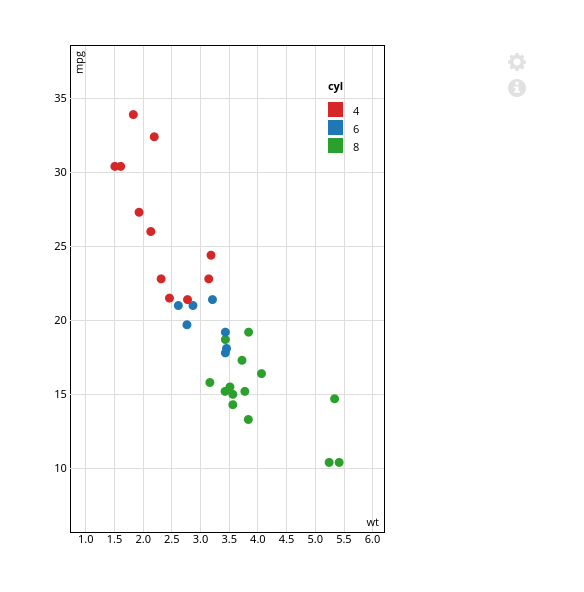## Custom tooltips

If the default tooltips don't suit your needs, you can customize them by providing a character vector to the `tooltip_text` argument. This can contain HTML tags for formatting.

``````tooltips <- paste("This is an incredible <strong>", rownames(mtcars),"</strong><br />with ",
mtcars\$cyl, "cylinders !")
scatterD3(data = mtcars, x = wt, y = mpg, tooltip_text = tooltips)
``````You can also disable tooltips entirely with `tooltips = FALSE`.

## Open URLs when clicking points

With the `url_var` argument, you can specify a character vectors of URLs, associated to each point, and which will be opened when the point is clicked.

``````mtcars\$urls <- paste0("https://www.duckduckgo.com/?q=", rownames(mtcars))
scatterD3(data = mtcars, x = wt, y = mpg, lab = names, url_var = urls)
``````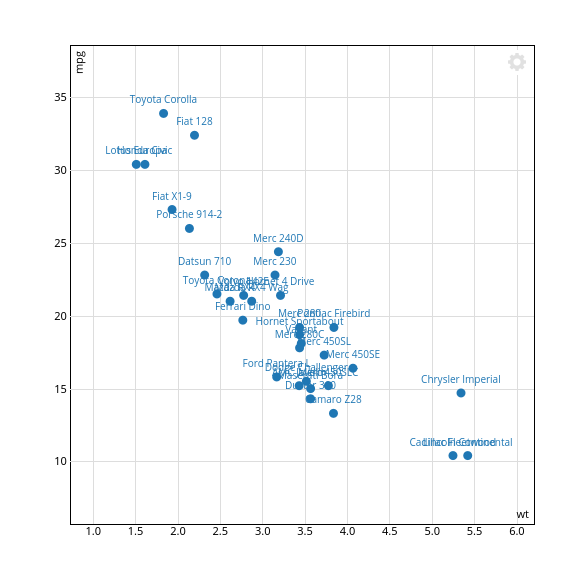Note that this won't work inside RStudio's internal browser.

## JavaScript callback on clicking point

The optional `click_callback` argument is a character string defining a JavaScript function to be called when a dot is clicked. It must accept two arguments : `html_id` (the unique `id` of the current scatterplot), and `i` (the index of the clicked point).

``````scatterD3(data = mtcars, x = wt, y = mpg,
click_callback = "function(id, index) {
alert('scatterplot ID: ' + id + ' - Point index: ' + index)
}")
``````One usage can be to pass the index of the clicked point back to Shiny when `scatterD3` is run inside a Shiny app. The following implementation can do it by using `Shiny.onInputChange()` :

``````scatterD3(data = mtcars, x = wt, y = mpg,
click_callback = "function(id, index) {
if(id && typeof(Shiny) != 'undefined') {
Shiny.onInputChange('selected_point', index);
}
}")
``````

You could then add something like this in your Shiny app `ui` :

``````textOutput("click_selected")
``````

And this in `server` :

``````output\$click_selected <- renderText(paste0("Clicked point : ", input\$selected_point))
``````

Thanks to detule and harveyl888 for the code.

Note that `url_var` and `click_callback` cannot be used at the same time.

## JavaScript zoom callback

The optional `zoom_callback` argument is a character string defining a JavaScript function to be called when a zoom event is triggered. It must accept two arguments `xmin`, `xmax`, `ymin` and `ymax` (in this order), which give the new `x` and `y` domains after zooming.

``````scatterD3(data = mtcars, x = wt, y = mpg,
zoom_callback = "function(xmin, xmax, ymin, ymax) {
var zoom = '<strong>Zoom</strong><br />xmin = ' + xmin + '<br />xmax = ' + xmax + '<br />ymin = ' + ymin + '<br />ymax = ' + ymax;
document.getElementById('zoomExample').innerHTML = zoom;
}")
``````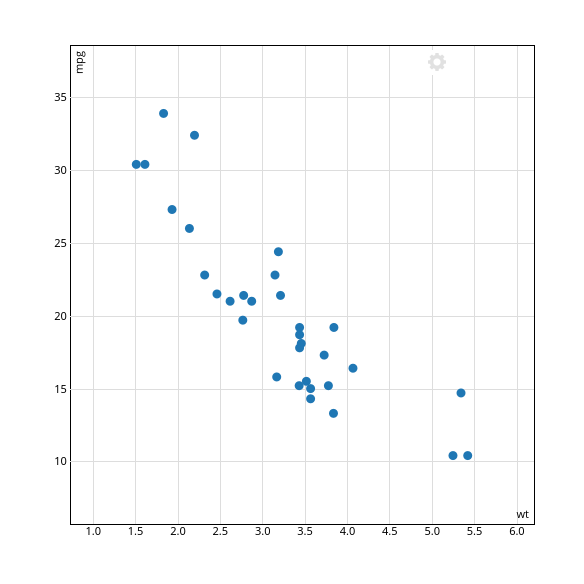Zoom
None yet !

## Confidence ellipses

You can draw a confidence ellipse around the points :

``````scatterD3(data = mtcars, x = wt, y = mpg, ellipses = TRUE)
``````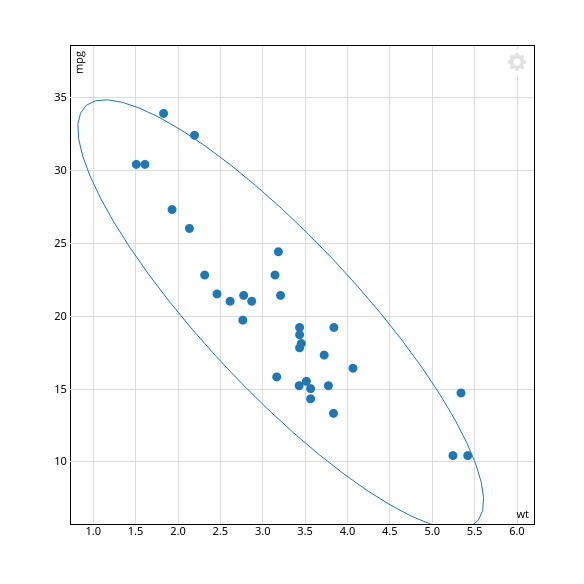Or around the different groups of points defined by `col_var` :

``````scatterD3(data = mtcars, x = wt, y = mpg, col_var = cyl, ellipses = TRUE)
``````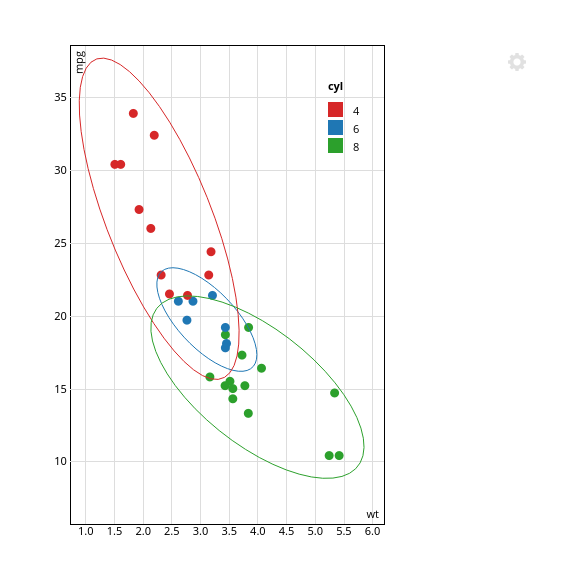Ellipses are computed by the `ellipse.default()` function of the ellipse package. The confidence level can be changed with the `ellipse_level` argument (`0.95` by default).

The “gear menu” is a small menu which can be displayed by clicking on the “gear” icon on the top-right corner of the plot. It allows to reset the zoom, export the current graph to SVG, and toggle lasso selection.

It is displayed by default, but you can hide it with the `menu = FALSE` argument.

``````scatterD3(data = mtcars, x = wt, y = mpg, menu = FALSE)
``````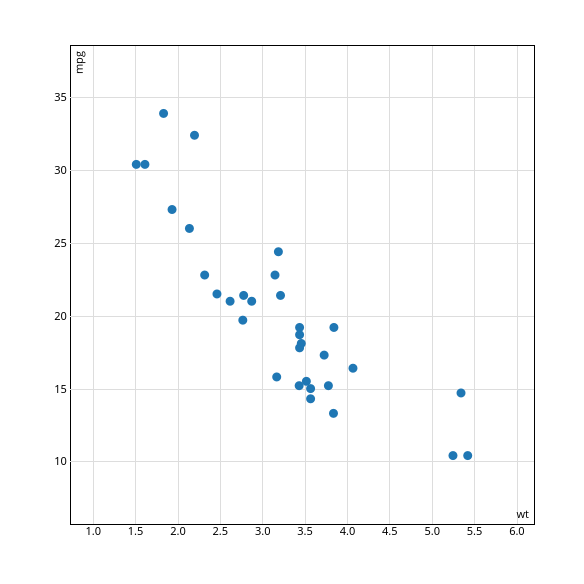## Lasso selection tool

Thanks to the d3-lasso-plugin integration made by @timelyportfolio, you can select and highlight points with a lasso selection tool. To activate it, just add a `lasso = TRUE` argument. The tool is used by shift-clicking and dragging on the plot area (if it doesn't activate, click on the chart first to give it focus).

``````mtcars\$names <- rownames(mtcars)
scatterD3(data = mtcars, x = wt, y = mpg, lab = names, lasso = TRUE)
``````To undo the selection, just shift-click again.

You can specify a custom JavaScript callback function to be called by passing it to the `lasso_callback` argument as a character string. This function should accept a `sel` argument, which is a d3 selection of selected points.

Here is an example which shows an alert with selected point labels :

``````mtcars\$names <- rownames(mtcars)
scatterD3(data = mtcars,
x = wt, y = mpg, lab = names,
lasso = TRUE,
lasso_callback = "function(sel) {alert(sel.data().map(function(d) {return d.lab}).join('\\n'));}")
``````## Custom labels positions export

The “gear menu” allows to export the current custom labels position as a CSV file for later reuse.

For example, if you change the labels placement in the following plot :

``````mtcars\$names <- rownames(mtcars)
scatterD3(data = mtcars, x = wt, y = mpg, lab = names)
``````You can then open the menu and select Export labels positions to save them into a CSV file. If you want to reuse these positions, you can use the `labels_positions` argument from `scatterD3` :

``````labels <- read.csv("scatterD3_labels.csv")
scatterD3(data = mtcars, x = wt, y = mpg, lab = names, labels_positions = labels)
``````

You can also use this file to reuse coordinates in a plot from a different package. The following example should work with `ggplot2` :

``````labels <- read.csv("scatterD3_labels.csv")
library(ggplot2)
ggplot() +
geom_point(data = mtcars, aes(x=wt, y=mpg)) +
geom_text(data = labels,
aes(x = lab_x,
y = lab_y,
label = lab))
``````

## Other options

Finally, and for more specific use cases, you can represent some points as an arrow starting from the origin by using the `type_var` argument, and you can add a unit circle with `unit_circle = TRUE`.

``````scatterD3(x = c(1, 0.9, 0.7, 0.2, -0.4, -0.5), xlab = "x",
y = c(1, 0.1, -0.5, 0.5, -0.6, 0.7), ylab = "y",
lab = LETTERS[1:6], type_var = c("point", rep("arrow", 5)),
unit_circle = TRUE, fixed = TRUE,
xlim = c(-1.2, 1.2), ylim = c(-1.2, 1.2))
``````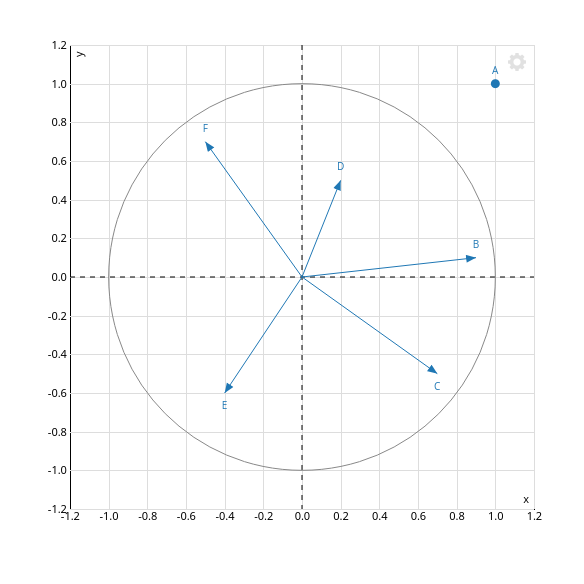## Shiny integration

### Transitions

Like every R HTML widget, shiny integration is straightforward. But as a D3 widget, `scatterD3` is updatable : changes in settings or data can be displayed via smooth transitions instead of a complete chart redraw, which can provide interesting visual clues.

For a small demonstration of these transitions, you can take a look at the sample scatterD3 shiny app.

Enabling transitions in your shiny app is quite simple, you just have to add the `transitions = TRUE` argument to your `scatterD3` calls in your shiny server code. There's only one warning : if your shiny application may filter on your dataset rows via a form control, then you must provide a `key_var` variable that uniquely and persistently identify your rows.

### Additional controls : Reset zoom and SVG export

Furthermore, `scatterD3` provides some additional handlers for three interactive features : SVG export, zoom resetting and lasso selection. Those are already accessible via the “gear menu”, but you may want to replace it with custom form controls.

By default, you just have to give the following `id` to the corresponding form controls :

• `#scatterD3-reset-zoom` : reset zoom to default on click
• `#scatterD3-svg-export` : link to download the currently displayed figure as an SVG file
• `#scatterD3-lasso-toggle` : toggle lasso selection

If you are not happy with these ids, you can specify their names yourself with the arguments `dom_id_svg_export`, `dom_id_reset_zoom` and `dom_id_toggle`.

### Sample app and source code

The sample scatterD3 shiny app allows you to see the different features described here. You can check its source code on GitHub for a better understanding of the different arguments.

## Known problems

Due to a lack of support of the `download` attribute in RStudio's interface, two problems may occur when exporting a plot to SVG, or labels positions to CSV :

• the file name suggested in the file save dialog is the data URI. You have to replace it with the name of your choice, and the correct extension (`.svg` or `.csv`).

• when replacing an existing file, if the data to be saved are shorter than the file, it seems that RStudio will just replace the beginning of the file with the new data, but keep existing file content at the end. A workaround is either to always save to a new file, or open the plot in a modern browser before exporting.